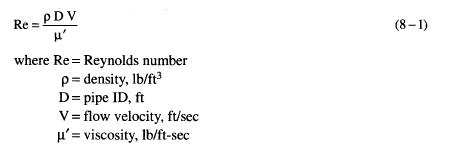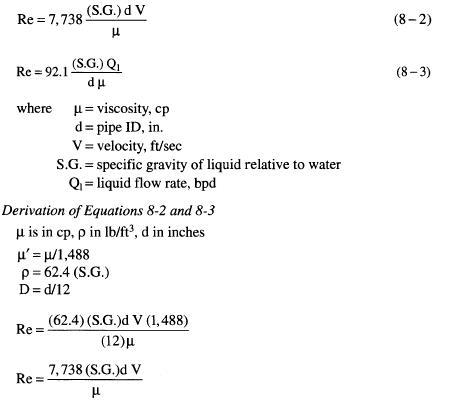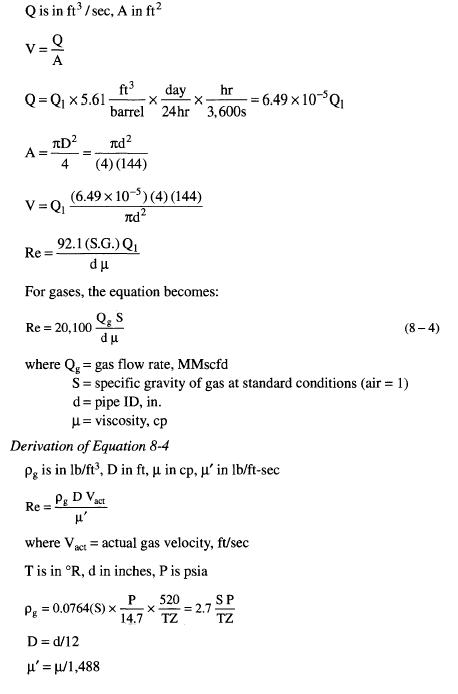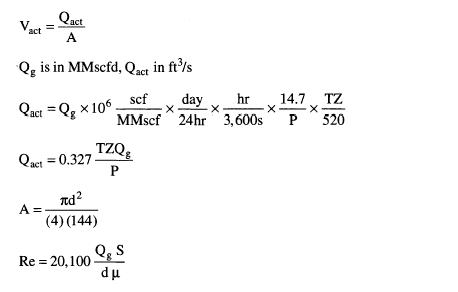﻿ Piping – Reynolds Number | Oil & Gas Process Engineering

## Piping – Reynolds Number

The Reynolds number is a dimensionless parameter that relates the ratio of inertial forces to viscous forces. It can be expressed by the following general equation:The Reynolds number can be expressed in more convenient terms. For liquids, the equation becomes: